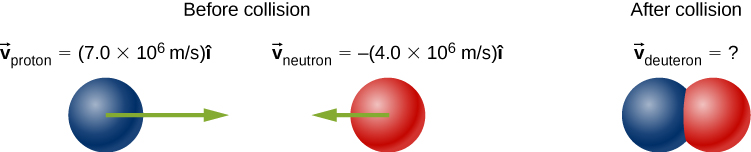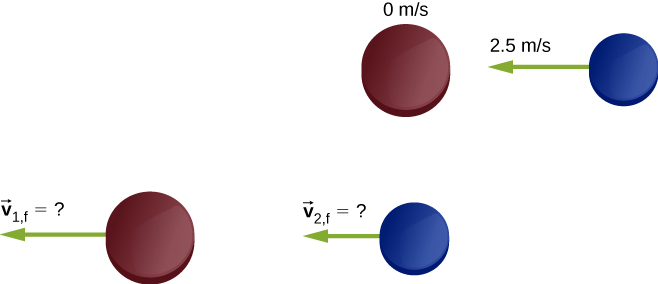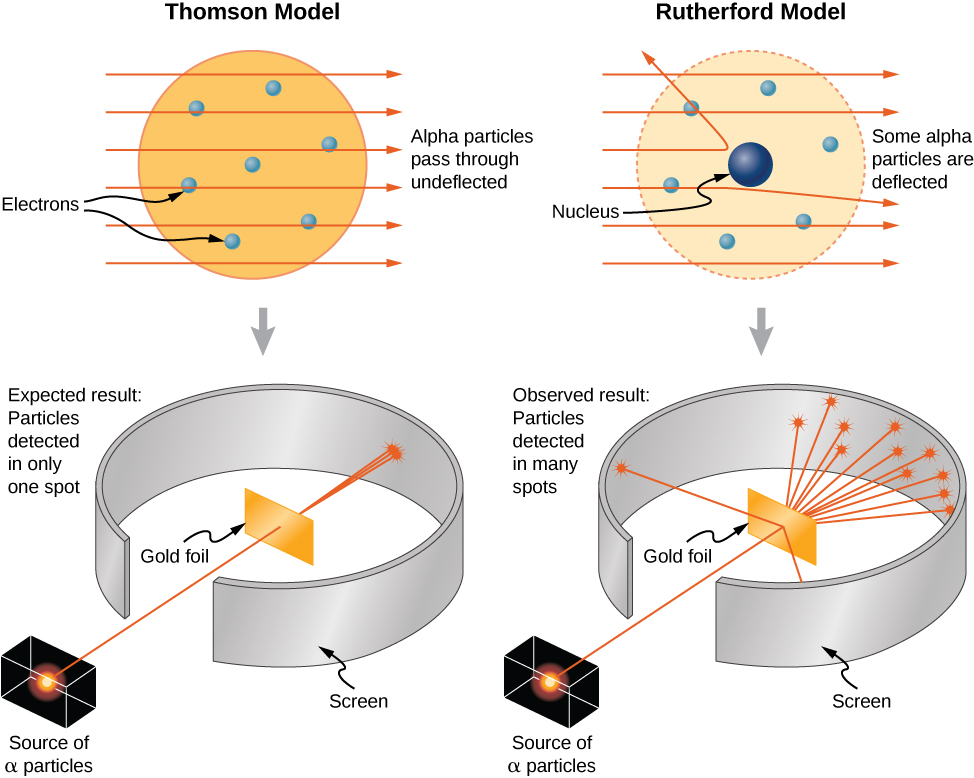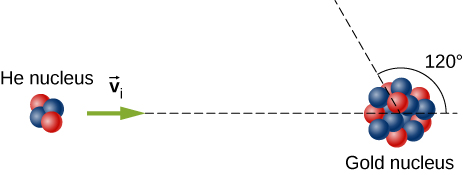9 Linear Momentum and Collisions

# 9.4 Types of Collisions

### Learning Objectives

By the end of this section, you will be able to:

• Identify the type of collision
• Correctly label a collision as elastic or inelastic
• Use kinetic energy along with momentum and impulse to analyze a collision

Although momentum is conserved in all interactions, not all interactions (collisions or explosions) are the same. The possibilities include:

• A single object can explode into multiple objects (one-to-many).
• Multiple objects can collide and stick together, forming a single object (many-to-one).
• Multiple objects can collide and bounce off of each other, remaining as multiple objects (many-to-many). If they do bounce off each other, then they may recoil at the same speeds with which they approached each other before the collision, or they may move off more slowly.

It’s useful, therefore, to categorize different types of interactions, according to how the interacting objects move before and after the interaction.

### One-to-Many

The first possibility is that a single object may break apart into two or more pieces. An example of this is a firecracker, or a bow and arrow, or a rocket rising through the air toward space. These can be difficult to analyze if the number of fragments after the collision is more than about three or four; but nevertheless, the total momentum of the system before and after the explosion is identical.

Note that if the object is initially motionless, then the system (which is just the object) has no momentum and no kinetic energy. After the explosion, the net momentum of all the pieces of the object must sum to zero (since the momentum of this closed system cannot change). However, the system will have a great deal of kinetic energy after the explosion, although it had none before. Thus, we see that, although the momentum of the system is conserved in an explosion, the kinetic energy of the system most definitely is not; it increases. This interaction—one object becoming many, with an increase of kinetic energy of the system—is called an explosion.

Where does the energy come from? Does conservation of energy still hold? Yes; some form of potential energy is converted to kinetic energy. In the case of gunpowder burning and pushing out a bullet, chemical potential energy is converted to kinetic energy of the bullet, and of the recoiling gun. For a bow and arrow, it is elastic potential energy in the bowstring.

### Many-to-One

The second possibility is the reverse: that two or more objects collide with each other and stick together, thus (after the collision) forming one single composite object. The total mass of this composite object is the sum of the masses of the original objects, and the new single object moves with a velocity dictated by the conservation of momentum. However, it turns out again that, although the total momentum of the system of objects remains constant, the kinetic energy doesn’t; but this time, the kinetic energy decreases. This type of collision is called inelastic.

In the extreme case, multiple objects collide, stick together, and remain motionless after the collision. Since the objects are all motionless after the collision, the final kinetic energy is also zero; the loss of kinetic energy is a maximum. Such a collision is said to be perfectly inelastic.

### Many-to-Many

The extreme case on the other end is if two or more objects approach each other, collide, and bounce off each other, moving away from each other at the same relative speed at which they approached each other. In this case, the total kinetic energy of the system is conserved. Such an interaction is called elastic.

In any interaction of a closed system of objects, the total momentum of the system is conserved $({\mathbf{\overset{\to }{p}}}_{f}={\mathbf{\overset{\to }{p}}}_{\text{i}})$ but the kinetic energy may not be:

• If $0 \lt {K}_{\text{f}} \lt {K}_{\text{i}}$, the collision is inelastic.
• If ${K}_{\text{f}}=0$, the collision is perfectly inelastic.
• If ${K}_{\text{f}}={K}_{\text{i}}$, the collision is elastic.
• If ${K}_{\text{f}} \gt {K}_{\text{i}}$, the interaction is an explosion.

The point of all this is that, in analyzing a collision or explosion, you can use both momentum and kinetic energy.

### Problem-Solving Strategy: Collisions

A closed system always conserves momentum; it might also conserve kinetic energy, but very often it doesn’t. Energy-momentum problems confined to a plane (as ours are) usually have two unknowns. Generally, this approach works well:

1. Define a closed system.
2. Write down the expression for conservation of momentum.
3. If kinetic energy is conserved, write down the expression for conservation of kinetic energy; if not, write down the expression for the change of kinetic energy.
4. You now have two equations in two unknowns, which you solve by standard methods.

### Example

#### Formation of a Deuteron

A proton (mass $1.67\times {10}^{-27}\,\text{kg}$) collides with a neutron (with essentially the same mass as the proton) to form a particle called a deuteron. What is the velocity of the deuteron if it is formed from a proton moving with velocity $7.0\times {10}^{6}\,\text{m/s}$ to the left and a neutron moving with velocity $4.0\times {10}^{6}\,\text{m/s}$ to the right?#### Strategy

Define the system to be the two particles. This is a collision, so we should first identify what kind. Since we are told the two particles form a single particle after the collision, this means that the collision is perfectly inelastic. Thus, kinetic energy is not conserved, but momentum is. Thus, we use conservation of energy to determine the final velocity of the system.

#### Solution

Treat the two particles as having identical masses M. Use the subscripts p, n, and d for proton, neutron, and deuteron, respectively. This is a one-dimensional problem, so we have

$M{v}_{\text{p}}-M{v}_{\text{n}}=2M{v}_{\text{d}}.$

The masses divide out:

$\begin{array}{ccc}\hfill {v}_{\text{p}}-{v}_{\text{n}}& =\hfill & 2{v}_{\text{d}}\hfill \\ \hfill 7.0\times {10}^{6}\,\text{m/s}-4.0\times {10}^{6}\,\text{m/s}& =\hfill & 2{v}_{\text{d}}\hfill \\ \hfill {v}_{\text{d}}& =\hfill & 1.5\times {10}^{6}\,\text{m/s.}\hfill \end{array}$

The velocity is thus ${\mathbf{\overset{\to }{v}}}_{\text{d}}=(1.5\times {10}^{6}\,\text{m/s})\mathbf{\hat{i}}$.

#### Significance

This is essentially how particle colliders like the Large Hadron Collider work: They accelerate particles up to very high speeds (large momenta), but in opposite directions. This maximizes the creation of so-called “daughter particles.”

### Example

#### Ice Hockey 2

(This is a variation of an earlier example.)

Two ice hockey pucks of different masses are on a flat, horizontal hockey rink. The red puck has a mass of 15 grams, and is motionless; the blue puck has a mass of 12 grams, and is moving at 2.5 m/s to the left. It collides with the motionless red puck (Figure). If the collision is perfectly elastic, what are the final velocities of the two pucks?Figure 9.20 Two different hockey pucks colliding. The top diagram shows the pucks the instant before the collision, and the bottom diagram show the pucks the instant after the collision. The net external force is zero.

#### Strategy

We’re told that we have two colliding objects, and we’re told their masses and initial velocities, and one final velocity; we’re asked for both final velocities. Conservation of momentum seems like a good strategy; define the system to be the two pucks. There is no friction, so we have a closed system. We have two unknowns (the two final velocities), but only one equation. The comment about the collision being perfectly elastic is the clue; it suggests that kinetic energy is also conserved in this collision. That gives us our second equation.

The initial momentum and initial kinetic energy of the system resides entirely and only in the second puck (the blue one); the collision transfers some of this momentum and energy to the first puck.

#### Solution

Conservation of momentum, in this case, reads

$\begin{array}{ccc}\hfill {p}_{\text{i}}& =\hfill & {p}_{\text{f}}\hfill \\ \hfill {m}_{2}{v}_{\text{2,i}}& =\hfill & {m}_{1}{v}_{\text{1,f}}+{m}_{2}{v}_{\text{2,f}}.\hfill \end{array}$

$\begin{array}{ccc}\hfill {K}_{\text{i}}& =\hfill & {K}_{\text{f}}\hfill \\ \hfill \frac{1}{2}{m}_{2}{v}_{\text{2,i}}^{2}& =\hfill & \frac{1}{2}{m}_{1}{v}_{\text{1,f}}^{2}+\frac{1}{2}{m}_{2}{v}_{\text{2,f}}^{2}.\hfill \end{array}$

There are our two equations in two unknowns. The algebra is tedious but not terribly difficult; you definitely should work it through. The solution is

$\begin{array}{ccc}\hfill {v}_{\text{1,f}}& =\hfill & \frac{({m}_{1}-{m}_{2}){v}_{\text{1,i}}+2{m}_{2}{v}_{\text{2,i}}}{{m}_{1}+{m}_{2}}\hfill \\ \hfill {v}_{2\text{f}}& =\hfill & \frac{({m}_{2}-{m}_{1}){v}_{\text{2,i}}+2{m}_{1}{v}_{\text{1,i}}}{{m}_{1}+{m}_{2}}.\hfill \end{array}$

Substituting the given numbers, we obtain

$\begin{array}{ccc}\hfill {v}_{\text{1,f}}& =\hfill & 2.22\,\frac{\text{m}}{\text{s}}\hfill \\ \hfill {v}_{\text{2,f}}& =\hfill & -0.28\,\frac{\text{m}}{\text{s}}.\hfill \end{array}$

#### Significance

Notice that after the collision, the blue puck is moving to the right; its direction of motion was reversed. The red puck is now moving to the left.

There is a second solution to the system of equations solved in this example (because the energy equation is quadratic): ${v}_{\text{1,f}}=-2.5\,\text{m/s},{v}_{\text{2,f}}=0$. This solution is unacceptable on physical grounds; what’s wrong with it?

This solution represents the case in which no interaction takes place: the first puck misses the second puck and continues on with a velocity of 2.5 m/s to the left. This case offers no meaningful physical insights.

### Example

#### Thor vs. Iron Man

The 2012 movie “The Avengers” has a scene where Iron Man and Thor fight. At the beginning of the fight, Thor throws his hammer at Iron Man, hitting him and throwing him slightly up into the air and against a small tree, which breaks. From the video, Iron Man is standing still when the hammer hits him. The distance between Thor and Iron Man is approximately 10 m, and the hammer takes about 1 s to reach Iron Man after Thor releases it. The tree is about 2 m behind Iron Man, which he hits in about 0.75 s. Also from the video, Iron Man’s trajectory to the tree is very close to horizontal. Assuming Iron Man’s total mass is 200 kg:

1. Estimate the mass of Thor’s hammer
2. Estimate how much kinetic energy was lost in this collision

#### Strategy

After the collision, Thor’s hammer is in contact with Iron Man for the entire time, so this is a perfectly inelastic collision. Thus, with the correct choice of a closed system, we expect momentum is conserved, but not kinetic energy. We use the given numbers to estimate the initial momentum, the initial kinetic energy, and the final kinetic energy. Because this is a one-dimensional problem, we can go directly to the scalar form of the equations.

#### Solution

1. First, we posit conservation of momentum. For that, we need a closed system. The choice here is the system (hammer + Iron Man), from the time of collision to the moment just before Iron Man and the hammer hit the tree. Let:
• ${M}_{\text{H}}=$ mass of the hammer
• ${M}_{\text{I}}=$ mass of Iron Man
• ${v}_{\text{H}}=$ velocity of the hammer before hitting Iron Man
• v$=$ combined velocity of Iron Man + hammer after the collision

Again, Iron Man’s initial velocity was zero. Conservation of momentum here reads:

${M}_{\text{H}}{v}_{\text{H}}=({M}_{\text{H}}+{M}_{\text{I}})v.$

We are asked to find the mass of the hammer, so we have

$\begin{array}{ccc}\hfill {M}_{\text{H}}{v}_{\text{H}}& =\hfill & {M}_{\text{H}}v+{M}_{\text{I}}v\hfill \\ \hfill {M}_{\text{H}}({v}_{\text{H}}-v)& =\hfill & {M}_{\text{I}}v\hfill \\ \hfill {M}_{\text{H}}& =\hfill & \frac{{M}_{\text{I}}v}{{v}_{\text{H}}-v}\hfill \\ & =\hfill & \frac{(200\,\text{kg})(\frac{2\,\text{m}}{0.75\,\text{s}})}{10\,\frac{\text{m}}{\text{s}}-(\frac{2\,\text{m}}{0.75\,\text{s}})}\hfill \\ & =\hfill & 73\,\text{kg.}\hfill \end{array}$

Considering the uncertainties in our estimates, this should be expressed with just one significant figure; thus, ${M}_{\text{H}}=7\times {10}^{1}\,\text{kg}$.

2. The initial kinetic energy of the system, like the initial momentum, is all in the hammer:
$\begin{array}{cc}\hfill {K}_{\text{i}}& =\frac{1}{2}{M}_{\text{H}}{v}_{\text{H}}^{2}\hfill \\ & =\frac{1}{2}(70\,\text{kg}){(10\,\text{m/s})}^{2}\hfill \\ & =3500\,\text{J.}\hfill \end{array}$

After the collision,

$\begin{array}{cc}\hfill {K}_{\text{f}}& =\frac{1}{2}({M}_{\text{H}}+{M}_{\text{I}}){v}^{2}\hfill \\ & =\frac{1}{2}(70\,\text{kg}+200\,\text{kg}){(2.67\,\text{m/s})}^{2}\hfill \\ & =960\,\text{J.}\hfill \end{array}$

Thus, there was a loss of $3500\,\text{J}-960\,\text{J}=2540\,\text{J}$.

#### Significance

From other scenes in the movie, Thor apparently can control the hammer’s velocity with his mind. It is possible, therefore, that he mentally causes the hammer to maintain its initial velocity of 10 m/s while Iron Man is being driven backward toward the tree. If so, this would represent an external force on our system, so it would not be closed. Thor’s mental control of his hammer is beyond the scope of this book, however.

### Example

#### Analyzing a Car Crash

At a stoplight, a large truck (3000 kg) collides with a motionless small car (1200 kg). The truck comes to an instantaneous stop; the car slides straight ahead, coming to a stop after sliding 10 meters. The measured coefficient of friction between the car’s tires and the road was 0.62. How fast was the truck moving at the moment of impact?

#### Strategy

At first it may seem we don’t have enough information to solve this problem. Although we know the initial speed of the car, we don’t know the speed of the truck (indeed, that’s what we’re asked to find), so we don’t know the initial momentum of the system. Similarly, we know the final speed of the truck, but not the speed of the car immediately after impact. The fact that the car eventually slid to a speed of zero doesn’t help with the final momentum, since an external friction force caused that. Nor can we calculate an impulse, since we don’t know the collision time, or the amount of time the car slid before stopping. A useful strategy is to impose a restriction on the analysis.

Suppose we define a system consisting of just the truck and the car. The momentum of this system isn’t conserved, because of the friction between the car and the road. But if we could find the speed of the car the instant after impact—before friction had any measurable effect on the car—then we could consider the momentum of the system to be conserved, with that restriction.

Can we find the final speed of the car? Yes; we invoke the work-kinetic energy theorem.

#### Solution

First, define some variables. Let:

• ${M}_{\text{c}}\,\text{and}\,{M}_{\text{T}}$ be the masses of the car and truck, respectively
• ${v}_{\text{T,i}}\,\text{and}{v}_{\text{T,f}}$ be the velocities of the truck before and after the collision, respectively
• ${v}_{\text{c,i}}\,\text{and}{v}_{\text{c,f}}$ Z be the velocities of the car before and after the collision, respectively
• ${K}_{\text{i}}\,\text{and}\,{K}_{\text{f}}$ be the kinetic energies of the car immediately after the collision, and after the car has stopped sliding (so ${K}_{\text{f}}=0$).
• d be the distance the car slides after the collision before eventually coming to a stop.

Since we actually want the initial speed of the truck, and since the truck is not part of the work-energy calculation, let’s start with conservation of momentum. For the car + truck system, conservation of momentum reads

$\begin{array}{c}{p}_{\text{i}}={p}_{\text{f}}\hfill \\ {M}_{\text{c}}{v}_{\text{c,i}}+{M}_{\text{T}}{v}_{\text{T,i}}={M}_{\text{c}}{v}_{\text{c,f}}+{M}_{\text{T}}{v}_{\text{T,f}}.\hfill \end{array}$

Since the car’s initial velocity was zero, as was the truck’s final velocity, this simplifies to

${v}_{\text{T,i}}=\frac{{M}_{\text{c}}}{{M}_{\text{T}}}{v}_{\text{c,f}}.$

So now we need the car’s speed immediately after impact. Recall that

$W=\Delta K$

where

$\begin{array}{cc}\hfill \Delta K& ={K}_{\text{f}}-{K}_{\text{i}}\hfill \\ & =0-\frac{1}{2}{M}_{\text{c}}{v}_{\text{c,f}}^{2}.\hfill \end{array}$

Also,

$W=\mathbf{\overset{\to }{F}}\cdot \mathbf{\overset{\to }{d}}=Fd\text{cos}\theta .$

The work is done over the distance the car slides, which we’ve called d. Equating:

$Fd\text{cos}\theta =-\frac{1}{2}{M}_{\text{c}}{v}_{\text{c,f}}^{2}.$

Friction is the force on the car that does the work to stop the sliding. With a level road, the friction force is

$F={\mu }_{\text{k}}{M}_{\text{c}}g.$

Since the angle between the directions of the friction force vector and the displacement d is $180^\circ$, and $\text{cos}(180^\circ)=–1,$ we have

$\text{−}({\mu }_{\text{k}}{M}_{\text{c}}g)d=-\frac{1}{2}{M}_{\text{c}}{v}_{\text{c,f}}^{2}$

(Notice that the car’s mass divides out; evidently the mass of the car doesn’t matter.)

Solving for the car’s speed immediately after the collision gives

${v}_{\text{c,f}}=\sqrt{2{\mu }_{\text{k}}gd}.$

Substituting the given numbers:

$\begin{array}{cc} \hfill {v}_{\text{c,f}}& =\sqrt{2(0.62)(9.81\,\frac{\text{m}}{{\text{s}}^{2}})(10\,\text{m})}\hfill \\ & =11.0\,\text{m/s}\text{.}\hfill \end{array}.$

Now we can calculate the initial speed of the truck:

${v}_{\text{T,i}}=(\frac{1200\,\text{kg}}{3000\,\text{kg}})(11.0\,\frac{\text{m}}{\text{s}})=4.4\,\text{m/s}\text{.}$

#### Significance

This is an example of the type of analysis done by investigators of major car accidents. A great deal of legal and financial consequences depend on an accurate analysis and calculation of momentum and energy.

Suppose there had been no friction (the collision happened on ice); that would make ${\mu }_{\text{k}}$ zero, and thus ${v}_{\text{c,f}}=\sqrt{2{\mu }_{\text{k}}gd}=0$, which is obviously wrong. What is the mistake in this conclusion?

If zero friction acts on the car, then it will continue to slide indefinitely ($d\to \infty$), so we cannot use the work-kinetic-energy theorem as is done in the example. Thus, we could not solve the problem from the information given.

### Subatomic Collisions and Momentum

Conservation of momentum is crucial to our understanding of atomic and subatomic particles because much of what we know about these particles comes from collision experiments.

At the beginning of the twentieth century, there was considerable interest in, and debate about, the structure of the atom. It was known that atoms contain two types of electrically charged particles: negatively charged electrons and positively charged protons. (The existence of an electrically neutral particle was suspected, but would not be confirmed until 1932.) The question was, how were these particles arranged in the atom? Were they distributed uniformly throughout the volume of the atom (as J.J. Thomson proposed), or arranged at the corners of regular polygons (which was Gilbert Lewis’ model), or rings of negative charge that surround the positively charged nucleus—rather like the planetary rings surrounding Saturn (as suggested by Hantaro Nagaoka), or something else?

The New Zealand physicist Ernest Rutherford (along with the German physicist Hans Geiger and the British physicist Ernest Marsden) performed the crucial experiment in 1909. They bombarded a thin sheet of gold foil with a beam of high-energy (that is, high-speed) alpha-particles (the nucleus of a helium atom). The alpha-particles collided with the gold atoms, and their subsequent velocities were detected and analyzed, using conservation of momentum and conservation of energy.

If the charges of the gold atoms were distributed uniformly (per Thomson), then the alpha-particles should collide with them and nearly all would be deflected through many angles, all small; the Nagaoka model would produce a similar result. If the atoms were arranged as regular polygons (Lewis), the alpha-particles would deflect at a relatively small number of angles.

What actually happened is that nearly none of the alpha-particles were deflected. Those that were, were deflected at large angles, some close to $180^\circ$—those alpha-particles reversed direction completely (Figure). None of the existing atomic models could explain this. Eventually, Rutherford developed a model of the atom that was much closer to what we now have—again, using conservation of momentum and energy as his starting point.Figure 9.21 The Thomson and Rutherford models of the atom. The Thomson model predicted that nearly all of the incident alpha-particles would be scattered and at small angles. Rutherford and Geiger found that nearly none of the alpha particles were scattered, but those few that were deflected did so through very large angles. The results of Rutherford’s experiments were inconsistent with the Thomson model. Rutherford used conservation of momentum and energy to develop a new, and better model of the atom—the nuclear model.

### Summary

• An elastic collision is one that conserves kinetic energy.
• An inelastic collision does not conserve kinetic energy.
• Momentum is conserved regardless of whether or not kinetic energy is conserved.
• Analysis of kinetic energy changes and conservation of momentum together allow the final velocities to be calculated in terms of initial velocities and masses in one-dimensional, two-body collisions.

### Conceptual Questions

Two objects of equal mass are moving with equal and opposite velocities when they collide. Can all the kinetic energy be lost in the collision?

Show Solution

Yes, all the kinetic energy can be lost if the two masses come to rest due to the collision (i.e., they stick together).

Describe a system for which momentum is conserved but mechanical energy is not. Now the reverse: Describe a system for which kinetic energy is conserved but momentum is not.

### Problems

A 5.50-kg bowling ball moving at 9.00 m/s collides with a 0.850-kg bowling pin, which is scattered at an angle to the initial direction of the bowling ball and with a speed of 15.0 m/s.

1. Calculate the final velocity (magnitude and direction) of the bowling ball.
2. Is the collision elastic?

a. 6.80 m/s, 5.33°; b. yes (calculate the ratio of the initial and final kinetic energies)

Ernest Rutherford (the first New Zealander to be awarded the Nobel Prize in Chemistry) demonstrated that nuclei were very small and dense by scattering helium-4 nuclei from gold-197 nuclei. The energy of the incoming helium nucleus was $8.00\times {10}^{-13}\,\text{J}$, and the masses of the helium and gold nuclei were $6.68\times {10}^{-27}\,\text{kg}$ and $3.29\times {10}^{-25}\,\text{kg}$, respectively (note that their mass ratio is 4 to 197).

a. If a helium nucleus scatters to an angle of $120^\circ$ during an elastic collision with a gold nucleus, calculate the helium nucleus’s final speed and the final velocity (magnitude and direction) of the gold nucleus.b. What is the final kinetic energy of the helium nucleus?

A 90.0-kg ice hockey player hits a 0.150-kg puck, giving the puck a velocity of 45.0 m/s. If both are initially at rest and if the ice is frictionless, how far does the player recoil in the time it takes the puck to reach the goal 15.0 m away?

Show Solution

2.5 cm

A 100-g firecracker is launched vertically into the air and explodes into two pieces at the peak of its trajectory. If a 72-g piece is projected horizontally to the left at 20 m/s, what is the speed and direction of the other piece?

In an elastic collision, a 400-kg bumper car collides directly from behind with a second, identical bumper car that is traveling in the same direction. The initial speed of the leading bumper car is 5.60 m/s and that of the trailing car is 6.00 m/s. Assuming that the mass of the drivers is much, much less than that of the bumper cars, what are their final speeds?

Show Solution

the speed of the leading bumper car is 6.00 m/s and that of the trailing bumper car is 5.60 m/s

Repeat the preceding problem if the mass of the leading bumper car is 30.0% greater than that of the trailing bumper car.

An alpha particle (4He) undergoes an elastic collision with a stationary uranium nucleus (235U). What percent of the kinetic energy of the alpha particle is transferred to the uranium nucleus? Assume the collision is one-dimensional.

Show Solution

6.6%

You are standing on a very slippery icy surface and throw a 1-kg football horizontally at a speed of 6.7 m/s. What is your velocity when you release the football? Assume your mass is 65 kg.

A 35-kg child sleds down a hill and then coasts along the flat section at the bottom, where a second 35-kg child jumps on the sled as it passes by her. If the speed of the sled is 3.5 m/s before the second child jumps on, what is its speed after she jumps on?

Show Solution

1.9 m/s

A boy sleds down a hill and onto a frictionless ice-covered lake at 10.0 m/s. In the middle of the lake is a 1000-kg boulder. When the sled crashes into the boulder, he is propelled over the boulder and continues sliding over the ice. If the boy’s mass is 40.0 kg and the sled’s mass is 2.50 kg, what is the speed of the sled and the boulder after the collision?

### Glossary

elastic
collision that conserves kinetic energy
explosion
single object breaks up into multiple objects; kinetic energy is not conserved in explosions
inelastic
collision that does not conserve kinetic energy
perfectly inelastic
collision after which all objects are motionless, the final kinetic energy is zero, and the loss of kinetic energy is a maximum